#Algebra 1 Worksheets

## Inequalities Worksheets

Here is a graphic preview for all of the Inequalities Worksheets. You can select different variables to customize these Inequalities Worksheets for your needs. The Inequalities Worksheets are randomly created and will never repeat so you have an endless supply of quality Inequalities Worksheets to use in the classroom or at home. We have properties of inequality handout, graphing single variables, one step by adding, subtracting, multiplying, and dividing, two step, multiple step, compound, and absolute value inequalities worksheets for your use.

Our Inequalities Worksheets are free to download, easy to use, and very flexible.

These Inequalities Worksheets are a good resource for students in the 5th Grade through the 8th Grade.

Click here for a Detailed Description of all the Inequalities Worksheets.

## Quick Link for All Inequalities Worksheets

Click the image to be taken to that Inequalities Worksheets.

##### Properties ofInequality Handout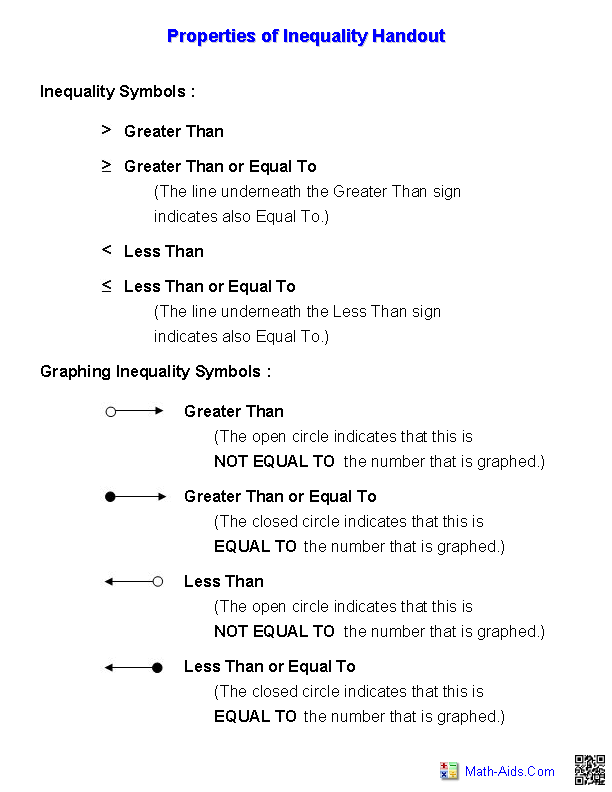##### Graphing Single VariableInequalities Worksheets##### One Step Inequalitiesby Adding and Subtracting##### One Step Inequalitiesby Multiplying and Dividing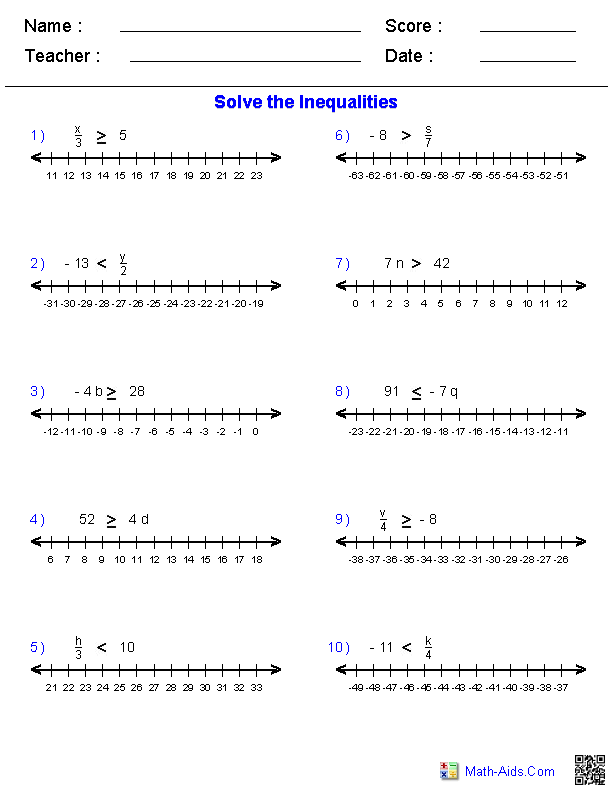##### One Step Inequalitiesby +, -, x, and ÷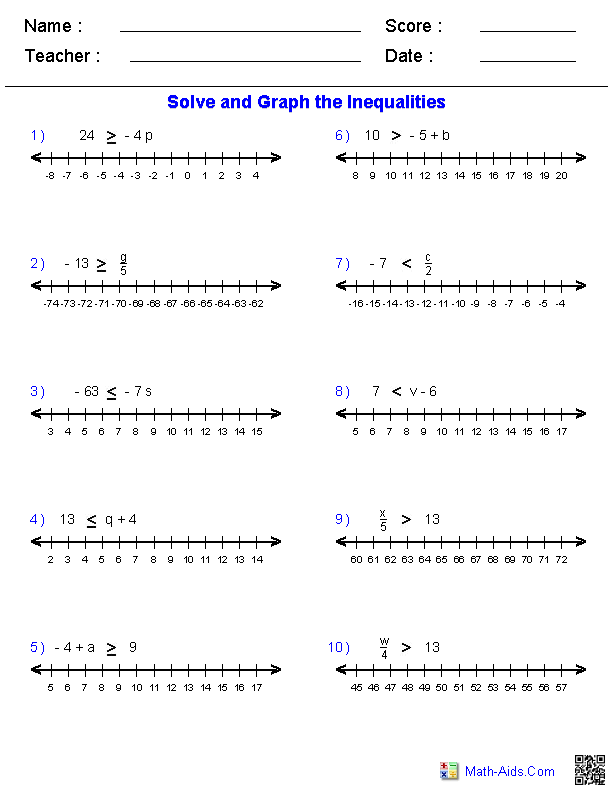##### Two Step InequalitiesInequalities Worksheets##### Multiple Step InequalitiesInequalities Worksheets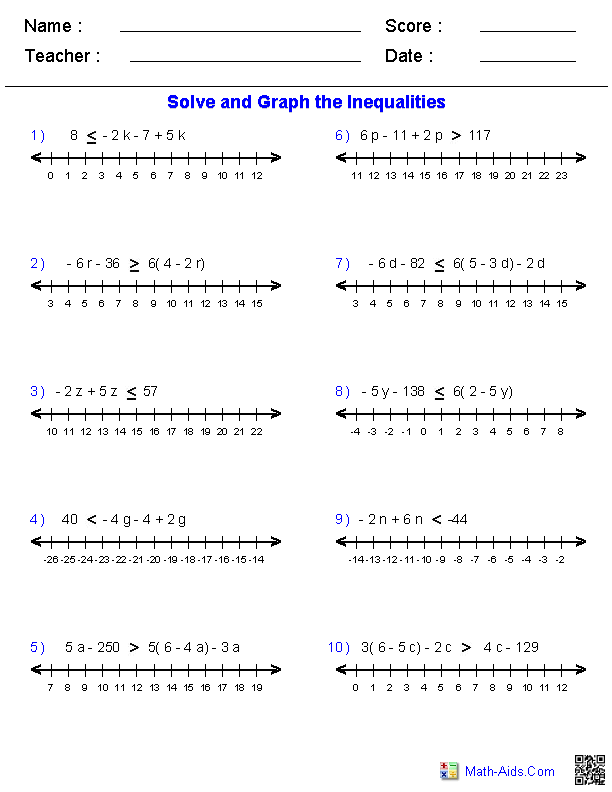##### Compound InequalitiesInequalities Worksheets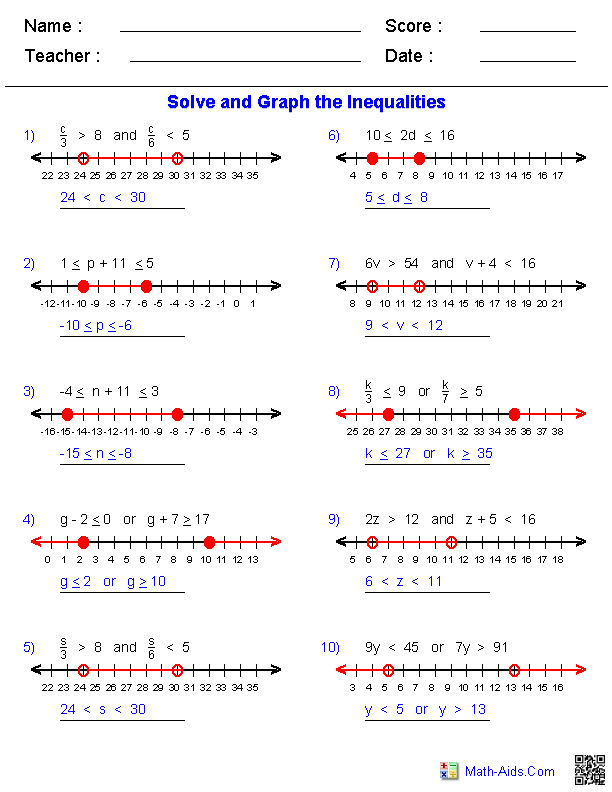##### Absolute Value InequalitiesInequalities WorksheetsRecommended Videos

## Detailed Description for All Inequalities Worksheets

Properties of Inequality Handout
This Inequality Worksheet will create a handout for the properties of inequalities. It explains the inequalities symbols, and graphing symbols with examples. This Inequality handout is a good resource for students in the 5th Grade through the 8th Grade.

Graphing Single Variable Inequalities Worksheets
These Inequality Worksheets will produce problems for graphing single variable inequalities. You may select which type of inequality and the type of numbers to use in the problems. You may choose to have the student to graph the inequalities, write the equation of the graphed inequality, or both. These Inequality Worksheets are a good resource for students in the 5th Grade through the 8th Grade.

One Step Inequalities by Adding and Subtracting
These Inequality Worksheets will produce graphing problems for one step inequalities by adding and subtracting. You may select which type of inequality to use in the problems. These Inequality Worksheets are a good resource for students in the 5th Grade through the 8th Grade.

One Step Inequalities by Multiplying and Dividing
These Inequality Worksheets will produce graphing problems for one step inequalities by multiplying and dividing. You may select which type of inequality to use in the problems. These Inequality Worksheets are a good resource for students in the 5th Grade through the 8th Grade.

One Step Inequalities by Adding, Subtracting, Multiplying, and Dividing
These Inequality Worksheets will produce graphing problems for one step inequalities by adding, subtracting, multiplying, and dividing. You may select which type of inequality to use in the problems. These Inequality Worksheets are a good resource for students in the 5th Grade through the 8th Grade.

Two Step Inequalities Worksheets
These Inequality Worksheets will produce two step inequalities problems for graphing. You may select which type of inequality to use in the problems. These Inequality Worksheets are a good resource for students in the 5th Grade through the 8th Grade.

Multiple Step Inequalities Worksheets
These Inequality Worksheets will produce multiple step inequalities problems for graphing. You may select which type of inequality to use in the problems. These Inequality Worksheets are a good resource for students in the 5th Grade through the 8th Grade.

Compound Inequalities Worksheets
These Inequality Worksheets will produce compound inequalities problems for graphing. You may select which type of inequality to use in the problems. These Inequality Worksheets are a good resource for students in the 5th Grade through the 8th Grade.

Absolute Value Inequalities Worksheets
These Inequality Worksheets will produce absolute value inequalities problems for graphing. You may select which type of inequality to use in the problems. These Inequality Worksheets are a good resource for students in the 5th Grade through the 8th Grade.